# CHAPTER 6 - Binomial Involving Calculus

The techniques of calculus enable us to sum a lot of series involving binomial coefficients. This is the subject of this section.
Suppose that we have to evaluate the sum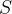$S$ given by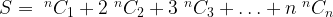$S = {\;^n}{C_1} + 2{\;^n}{C_2} + 3{\;^n}{C_3} + \ldots + n{\;^n}{C_n}$
From now on, to avoid clutter, we’ll write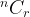$^n{C_r}$ as simply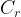${C_r}$, where the upper index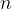$n$ should be understood to be present. Thus,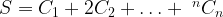$S = {C_1} + 2{C_2} + \ldots + {\;^n}{C_n}$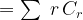$= \sum \;r\,{C_r}$
This series can be generated using a manipulation involving differentiation, as follows:
Consider the binomial expansion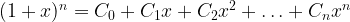${(1 + x)^n} = {C_0} + {C_1}x + {C_2}{x^2} + \ldots + {C_n}{x^n}$
If we differentiate both sides with respect to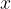$x$, look at what we’ll obtain: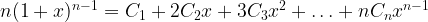$n{(1 + x)^{n - 1}} = {C_1} + 2{C_2}x + 3{C_3}{x^2} +\ldots + n{C_n}{x^{n - 1}}$
Now, all that remains is to substitute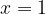$x = 1$, upon which we obtain:$n \cdot {2^{n - 1}} = {C_1} + 2{C_2} + 3{C_3} + \ldots + \;n\,{C_n}$
This is what we were looking for. Thus,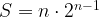$S = n \cdot {2^{n - 1}}$
Had we substituted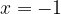$x = - 1$, we would’ve obtained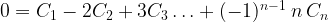$0 = {C_1} - 2{C_2} + 3{C_3}\ldots + {( - 1)^{n - 1}}\;n\,{C_n}$
Thus, we have evaluated another interesting sum.
Suppose that we now wish to evaluate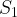${S_1}$ given by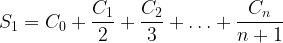${S_1} = {C_0} + \dfrac{{{C_1}}}{2} + \dfrac{{{C_2}}}{3} +\ldots + \dfrac{{{C_n}}}{{n + 1}}$
The alert reader would immediately realize that integration needs to be applied here. How exactly to do so is now described. Consider again the expansion.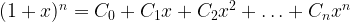${(1 + x)^n} = {C_0} + {C_1}x + {C_2}{x^2} +\ldots + {C_n}{x^n}$
If we integrate this with respect to$x$, between some limits say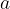$a$ to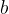$b$, we obtain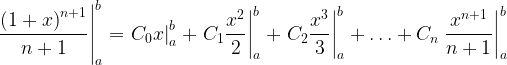$\left. {\dfrac{{{{(1 + x)}^{n + 1}}}}{{n + 1}}} \right|_a^b = \left. {{C_0}x} \right|_a^b + \left. {{C_1}\dfrac{{{x^2}}}{2}} \right|_a^b + \left. {{C_2}\dfrac{{{x^3}}}{3}} \right|_a^b + \ldots + {C_n}\left. {\dfrac{{{x^{n + 1}}}}{{n + 1}}} \right|_a^b$
To generate the sum${S_1}$ a little thought will show that we need to use$a = 0, b = 1$, so that we obtain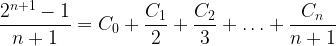$\dfrac{{{2^{n + 1}} - 1}}{{n + 1}} = {C_0} + \dfrac{{{C_1}}}{2} + \dfrac{{{C_2}}}{3} + \ldots + \dfrac{{{C_n}}}{{n + 1}}$
Thus,${S_1}$ equals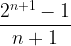$\dfrac{{{2^{n + 1}} - 1}}{{n + 1}}$
Try some other values for$a$ and$b$ and hence generate other series on your own. Be as varied as you can in choosing these limits.

### Related Posts

There is no other posts in this category.